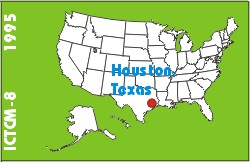# Exploring the Gini Index of Inequality with Derive

### Robert A. Leslie

Math Department
Agnes Scott College
Decatur, GA 30030
USA
Phone: (404) 638-6434
Fax: (404) 638-6177

 Click to access this paper: paper.pdf

## ABSTRACT

Suppose that X represents the per capita income of a certain region, with probability density function f(x) for x0 and F(x), the cumulative distribution function of X, for x0. For each number a in [0,1] the function g(a) is defined as that fraction of total regional income which is attributable to the poorest ( 100alpha)% of the given population. For example, it might be that the poorest 40% of the population garners only 15% of the income.

Keyword(s): Derive, statistics